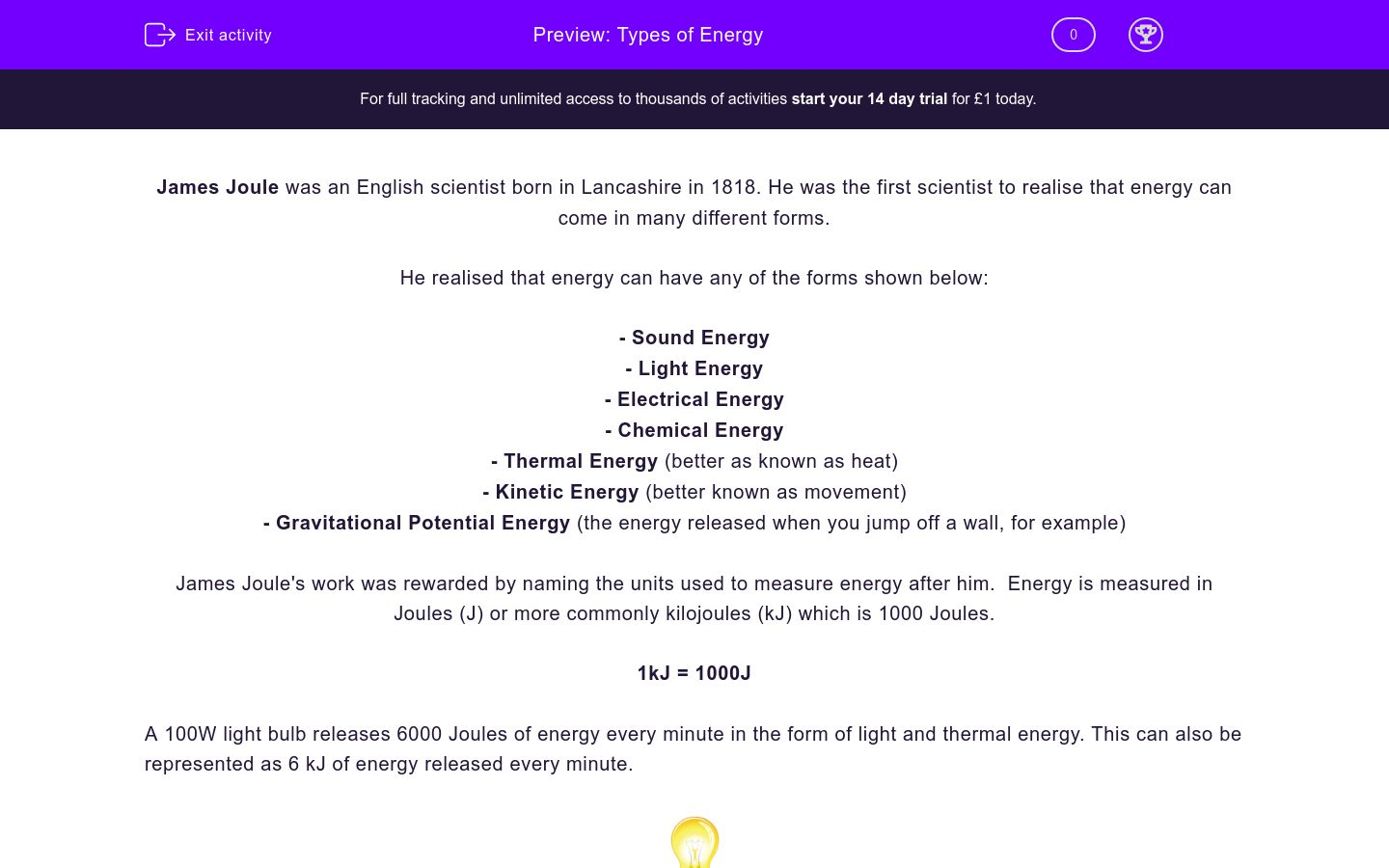# Types of Energy

In this worksheet, students will look at all the various forms of energy.Key stage:  KS 3

Curriculum topic:   Physics: Energy

Curriculum subtopic:   Energy Changes and Transfers

Difficulty level:### QUESTION 1 of 10

James Joule was an English scientist born in Lancashire in 1818. He was the first scientist to realise that energy can come in many different forms.

He realised that energy can have any of the forms shown below:

- Sound Energy

- Light Energy

- Electrical Energy

- Chemical Energy

- Thermal Energy (better as known as heat)

- Kinetic Energy (better known as movement)

- Gravitational Potential Energy (the energy released when you jump off a wall, for example)

James Joule's work was rewarded by naming the units used to measure energy after him.  Energy is measured in Joules (J) or more commonly kilojoules (kJ) which is 1000 Joules.

1kJ = 1000J

A 100W light bulb releases 6000 Joules of energy every minute in the form of light and thermal energy. This can also be represented as 6 kJ of energy released every minute.Which of the following are not types of energy?

Kinetic

Thermal

Heat

Time

Sound

Smell

What was the name of the Scientist who was the first to realise that there are many forms of energy?

Albert Einstein

James Joule

Isaac Newton

Brian Cox

What are the units used to measure energy?

Joules (J)

Seconds (s)

Decibels (db)

Look at the sentence below. Tick two words from the list below which fill in the gaps. Use the clues to help you!

There are many forms of energy. ___________ (movement) energy and __________ (heat) energy are just two examples.

sound

kinetic

chemical

thermal

electricalWhat is the correct name for the type of energy released when a raindrop falls from the sky?

Thermal

Gravitational potential

Kinetic

Energy is measured in Joules. What number of Joules is represented by the symbol kJ?

1 J

100 J

1000 J

Shown below is the label from a popular type of food.By looking at the picture, work out how many Joules of energy are contained in 100g of the food.

1570 J

15700 J

157000 J

1570000 J

A 12V battery releases approximetly 3600 J of energy every minute. What is 3600 J of energy in kiloJoules?

3.6 kJ

36 kJ

360 kJ

• Question 1

Which of the following are not types of energy?

Time
Smell
EDDIE SAYS
There are many different types, but time and smell cannot be classed as energy.
• Question 2

What was the name of the Scientist who was the first to realise that there are many forms of energy?

James Joule
EDDIE SAYS
These are all famous Scientists, but it was James Joule who first realised that there are many different forms of energy. Albert Einstein and Isaac Newton are very famous Physicists and Brian Cox is a modern Physicist who presents lots of programs about science on television - he even had a number one hit in the 1990's! Who said Scientists aren't cool?
• Question 3

What are the units used to measure energy?

Joules (J)
EDDIE SAYS
Again, Joules are named after the Scientist James Joule.
• Question 4

Look at the sentence below. Tick two words from the list below which fill in the gaps. Use the clues to help you!

There are many forms of energy. ___________ (movement) energy and __________ (heat) energy are just two examples.

kinetic
thermal
EDDIE SAYS
The proper name for movement is kinetic energy. Thermal energy is another term for heat. It is important to learn all the types of energy listed in the introduction.
• Question 5What is the correct name for the type of energy released when a raindrop falls from the sky?

Gravitational potential
EDDIE SAYS
The force of gravity pulls the water drops to the ground. The higher up an object or substance is the more gravitational potential energy it has. This energy is transferred to kinetic energy as it falls. What happens to the energy when it hits your window? Hmmm...
• Question 6

Energy is measured in Joules. What number of Joules is represented by the symbol kJ?

1000 J
EDDIE SAYS
kJ stands for kiloJoule and 1 kiloJoule equals 1000 Joules of energy.
• Question 7

Shown below is the label from a popular type of food.By looking at the picture, work out how many Joules of energy are contained in 100g of the food.

1570000 J
EDDIE SAYS
If 1 kJ is equal to 1000 J, then 1570 kJ is equal to 1570000 J (1570 x 1000), which seems like a lot but think about the amount of energy it took you to work out the answer to that difficult question!
• Question 8

A 12V battery releases approximetly 3600 J of energy every minute. What is 3600 J of energy in kiloJoules?

3.6 kJ
EDDIE SAYS
This is another tricky one. To convert Joules into kiloJoules, divide by 1000.
3600 ÷ 1000 = 3.6 kJ
---- OR ----